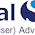## Friday, September 23, 2016

### Debt Equity Ratio - Formula

#### Debt Equity Ratio Formula:Debt Equity Ratio Formula

#### Meaning:

Debt Equity Ratio measures the firm's ability to meet its long-term obligations on time, by comparing with the firm's own money or net worth. As a measure of additional safety, value of intangible assets like goodwill and brands are deducted from the net worth.

#### Example:Example Calculation of Debt Equity Ratio of NMDC Ltd.

#### Required Number:

The Debt Equity Ratio must be one or below. Lower the number than one so much stronger the company is and vise-versa.

1.2.